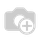• Prezzi

# How can I calculate the cost of a product [v13]

How can I calculate the cost of a product based on the following formula

cost = Purchase price + the cost of storage in warehouse A (warehouse price / day * number of days of storage of each product) + the cost of transit to PoS + the cost of storage in the PoS (PoS price / day * number of days of storage of each product)

thank you in advance